# Infinite Series

## infinite series

[′in·fə·nət ′sir·ēz]
(mathematics)
An indicated sum of an infinite sequence of quantities, written a1+ a2+ a3+ ⋯, or
McGraw-Hill Dictionary of Scientific & Technical Terms, 6E, Copyright © 2003 by The McGraw-Hill Companies, Inc.
The following article is from The Great Soviet Encyclopedia (1979). It might be outdated or ideologically biased.

## Infinite Series

an infinite sum of, for example, the form

ul + u2 + u3 + … + un+

or, more concisely, (1)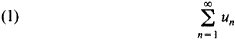A simple example of an infinite series encountered in elementary mathematics is the sum of a decreasing geometric progression:

(2) 1 + q + q2 ++ (qn += 1/(1 - q) ǀqǀ< 1

Often called simply series, infinite series are extensively used in mathematics and its applications both in theoretical studies and in approximate numerical solutions of problems.

Many numbers can be expressed in the form of special infinite series that permit easy calculation of the approximate values of the numbers to the required degree of accuracy. For example, the number πcan be computed from the series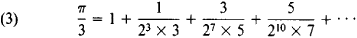For the base e of natural logarithms there exists the series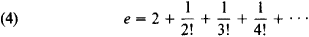The value of the natural logarithm ln 2 can be obtained from the series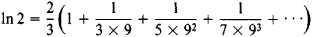Expansion in infinite series is a powerful technique for studying functions. Series expansions are used, for example, to calculate approximate values of functions, to calculate and estimate the values of integrals, and to solve algebraic, differential, and integral equations.

When in numerical calculations we replace an infinite series by the sum of the initial terms of the series, it is useful to have an estimate of the resultant error. This gives us an estimate of the rate of convergence of the series. Moreover, it is desirable to use series for which these errors tend to zero rapidly as the number n of terms increases. For example, in the case of series (4) the error estimate has the form 0 < e - sn<1/n!n.

A quantity can be expressed by different series. Thus, series (3) is not the only series for the number 77;an example of another series is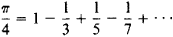This series, however, converges much more slowly than the series (3) and is therefore not convenient for the approximate calculation of π. The rate of convergence of a series can sometimes be increased through the use of certain transformations of the series.

Not all properties of finite sums carry over to infinite sums. For example, if we take the series

(5) 1 - 1 + 1 - 1 + …

and group its terms in succession by twos, we obtain (1–1) + (l-l) + … = 0; a different result is obtained when we group the terms as follows: 1 - (1 - 1) - (1 - 1) - … = 1. We therefore need a precise definition of an infinite sum, and, having defined this concept, we must verify whether the laws established for finite sums are valid for infinite sums. It can be proved that, under certain conditions, such principles as the commutative and associative laws of addition, the distributive property of multiplication with respect to addition, and the rules of term-by-term differentiation and integration are preserved for sums with an infinite number of terms.

Series of numbers. Series (1) can be formally defined as a pair of sequences of real or complex numbers {un} and {sn} such that sn = u1 + … + un, n = 1, 2, …. The first sequence is called the sequence of terms of the series, and the second is called the sequence of partial sums of the series; more precisely, sn is called the nth partial sum of series (1). Series (1) is said to be convergent if the sequence of its partial sums {sn} converges. In this case the limit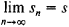is called the sum of the series, and we write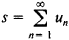Thus, expression (1) is used both for the series and, if the series converges, for the sum of the series. If the sequence of partial sums has no limit, then the series is said to be divergent. Series (2) is an example of a convergent series, and series (5) is an example of a divergent series. Every series uniquely defines the sequence of its partial sums. The converse statement is also true: for any sequence {sn} there exists a unique series for which this sequence is the sequence of partial sums of the series; the terms un of the series are defined by the formulas u1 = s1…,

un+1 = sn+1 sn, …, n= 1, 2, Consequently, the study of series is equivalent to the study of sequences.

The series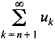is called the remainder after n terms of series (1). If a series converges, then all its remainders converge; if some remainder of a series converges, then the series itself also converges. If the remainder after n terms of series (1) converges and the sum of the remainder is equal to rn, then s = sn + rn.

If series (1) and the series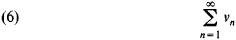converge, then the series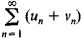also converges. This series is called the sum of series (1) and series (6), and its value is equal to the sum of these series. If series (1) converges and λ is a complex number, then the product of the series and λ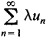also converges. Moreover,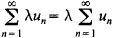The Cauchy condition for convergence of a series does not use the concept of the sum of a series. This is of great importance because, for example, the sum of the series may be unknown. According to the Cauchy condition, in order for series (1) to converge, it is necessary and sufficient that, for any ∊ > 0, there exist a number n such that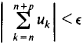for all n > n and all integers ρ≥ 0. It follows that if series (1) converges, then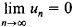The converse is not true: the nth term of the harmonic series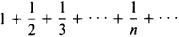tends toward zero, although this series diverges.

Series with nonnegative terms play an important role in the theory of series. In order for such a series to converge, it is necessary and sufficient that the sequence of its partial sums be bounded above. If, however, the series diverges, then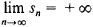We therefore write in this case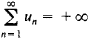A number of tests for convergence exist for series with non-negative terms.

According to the integral test for convergence, if the function f(x) is defined for all x≥ 1, is nonnegative, and decreasing, then the series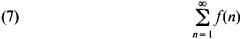converges if, and only if, the integral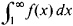converges. Through the use of this test we can easily establish that the series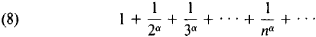converges when α > 1 and diverges when α ≤ 1.

Convergence can also be verified by the comparison test: if for any two series (1) and (6) with nonnegative terms there exists a constant c > 0 such that 0 ≤ un ≤ cvn, then the convergence of series (6) implies the convergence of series (1), and the divergence of series (1) implies the divergence of series (6). Series (8) is usually selected for comparison, and in the given series the principal part of the form A/nα is singled out. By using this method we can immediately demonstrate the convergence of the series with the nth term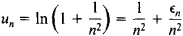where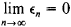This series converges because the series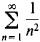converges.

The following rule is a corollary of the comparison test: if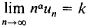then the series converges when α > 1 and 0 ≤ k < + ∞ and diverges when α ≤ 1 and 0 < k≤ + ∞. Thus, the series with the nth term un = sin (1/n2) converges, because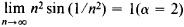The series with un= tan (π /n), on the other hand, diverges; here,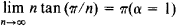Two corollaries of the comparison test frequently prove useful. One is d’ Alembert’s test: if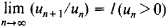exists, then series (1) converges when 1 < 1 and diverges when 1 > 1. The other is Cauchy’s root test: if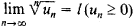exists, then series (1) converges when 1 < 1 and diverges when 1 > 1. Both in the case of d’ Alembert’s test and in the case of Cauchy’s test the series can be divergent or convergent when 1 = 1.

Absolutely convergent series constitute an important class of infinite series. Series (1) is said to be absolutely convergent if the series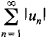converges.

If a series converges absolutely, then it also converges in the ordinary sense. The series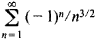converges absolutely; the series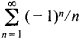however, converges only in the ordinary sense. The sum of absolutely convergent series and the product of an absolutely convergent series and a number are also absolutely convergent series. The properties of finite sums are carried over most completely to absolutely convergent series. Suppose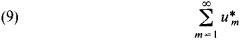is a series whose terms are the same as those of series (1) but are in general arranged in a different order. If series (1) converges absolutely, then series (9) also converges and has the same sum as series (1). If series (1) and (6) converge absolutely, then the series obtained from all possible products umvn of these series’ terms, arranged in an arbitrary order, also converges absolutely. Moreover, if the sum of the series is equal to s and the sums of series (1) and (6) are equal to s1 and s2, respectively, then s = s1s2; in other words, absolutely convergent series can be multiplied term by term without concern for the order of the terms. The convergence test for series with nonnegative terms can be used to establish the absolute convergence of series.

Convergent series that do not converge absolutely are said to be conditionally convergent. The sums of such series are not independent of the order of the terms. According to Riemann’s theorem, by an appropriate rearrangement of the terms of a given conditionally convergent series we can obtain a divergent series or a series that has a prescribed sum. The series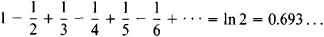is an example of a conditionally convergent series. If the terms of this series are rearranged so that two positive terms are followed by one negative term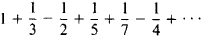then the sum of the series will be increased by a factor of 1.5. Several tests for convergence exist that are applicable to conditionally convergent series. An example is Leibniz’ test: if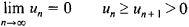then the alternating series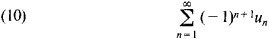converges. Through the use of, for example, Abel’s transformation more general tests can be obtained for series representable in the form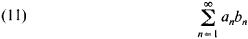One such test is Abel’s test: if the sequence {an} is monotonie and bounded and the series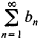converges, then series (11) also converges. According to Dirichlet’s test, if the sequence {an} tends monotonically to zero and the sequence of the partial sums of the series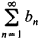is bounded, then series (11) converges. For example, by applying Dirichlet’s test we can show that the series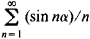converges for all real α.

Series of the form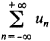are sometimes considered. Such a series is said to be convergent if the series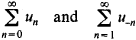converge. The sum of these series is called the sum of the original series.

Multiple series have a more complex structure. These are series of the form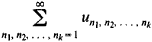where the

un1, n2,..., nk

are given—in general, complex—numbers indexed by k subscripts n1, n2, …, n2, each of which varies independently over the natural numbers. The simplest series of this type are double series.

For some series of numbers it is possible to obtain simple formulas for the value of the remainder or for an estimate of the remainder. These formulas are extremely important, for example, in evaluating the accuracy of calculations made with series. Thus, the remainder for the sum of geometric progression (2) is

rn = qn+1/(a – q) ǀqǀ < 1

For series (7), under the assumptions made, we have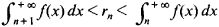For series (10) we have the formula

ǀrnǀ ≤ un+1

By using certain special transformations we can sometimes improve the convergence of a convergent series. Both convergent series and divergent series are used in mathematics. In the case of divergent series, more general concepts of the sum of a series are introduced. For example, divergent series (5) can be summed in a certain way to ½.

Series of functions. The concept of an infinite series can be extended in a natural way to the case where the terms of the series are the functions un = un (x) defined on some set E; these can be real functions, complex functions, or, more generally, functions whose values belong to some metric space. The series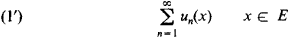in this case is said to be a series of functions.

If series (1’) converges at every point of E, then it is said to be convergent on E. Thus the series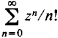converges throughout the complex plane. The sum of a convergent series of functions that are continuous, for example, on some closed interval is not necessarily a continuous function. The properties of continuity, differentiability, and integrability of finite sums of functions carry over to series of functions under certain conditions that are formulated in terms of uniform convergence of series. Convergent series (1’) is said to be uniformly convergent on E if, for all points in E and for sufficiently large n, the deviation of the partial sums of the series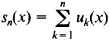from the sum of the series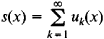does not exceed the same arbitrarily small quantity. More precisely, the series is uniformly convergent if, for arbitrary ∊ > 0, there exists an n such that

ǀs(x) – sn(x)ǀ ≤ ∊

for all n ≥ n and for all points x ∊ E. This condition is equivalent to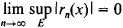where rn(x) = s(x) – sn(x) and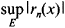is the least upper bound of Irn (x) I on E. For example, the series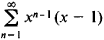converges uniformly on the closed interval [0, q] when 0 < q < 1 but does not converge uniformly on the closed interval [0, 1].

Cauchy’s condition can be stated as follows: in order for series (1’) to converge uniformly on E, it is necessary and sufficient that, for any ∊ > 0, there exists an n such that

ǀu(x) + … +un+p(xǀ < ∊

for all n ≥ n all ρ≥ 0, and all points xE.

According to Weierstrass’ test, if there exists a convergent series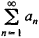such that ǀun(x)ǀ ≤ an, xE, n = 1, 2, …, then series (1’) converges uniformly on E.

The sum of a uniformly convergent series of functions that are continuous on some closed interval (or, more generally, on some topological space) is a continuous function on this interval (on this space). The sum of a uniformly convergent series of functions integrable on some set is an integrable function on this set, and the series can be integrated term by term. If the sequence of partial sums of a series of integrable functions converges in the mean to some integrable function, then the integral of this function is equal to the sum of the series of integrals of the terms of the series. Integrability here is construed in the sense of Riemann or Lebesgue. For Lebesgue integrable functions, a series with an almost everywhere convergent sequence of partial sums can be integrated term by term if we have a uniform estimate of the partial sums’ absolute values by some Lebesgue integrable function. Suppose series (1’) is convergent on some closed interval; if the terms of the series are difierentiable on the interval and if the series of their derivatives converges uniformly, then the sum of the original series is also differentible on this interval, and the series can be differentiated term by term.

The concept of a series of functions can also be generalized to the case of multiple series. In different branches of mathematics and its applications, extensive use is made of the expansions of functions in series of functions, especially in power series, trigonometric series, and, more generally, series of eigenfunctions of some operators.

History. The concept of infinite sums was arrived at long ago by the mathematicians of ancient Greece, who made use of the sum of terms of an infinite geometric progression with positive denominators less than unity. The infinite series entered mathematics as an independent concept in the 17th century. Newton and Leibniz systematically used infinite series to solve both algebraic and differential equations. The formal theory of infinite series was developed in the 18th and 19th centuries in the works of such mathematicians as Jakob Bernoulli, Johann Bernoulli, B. Taylor, C. Maclaurin, L. Euler, J. d’ Alembert, and J. Lagrange. Both convergent and divergent series were used in this period, but the question of the legitimacy of operations on them was not fully clarified. A rigorous theory of series, based on the concept of limits, was developed in the 19th century in the works of, for example, K. Gauss, B. Bolzano, A. Cauchy, P. Dirichlet, N. Abel, K. Weierstrass, and G. Riemann.

### REFERENCES

Markushevich, A. I. Riady: Elementarnyi ocherk, 3rd ed. Moscow, 1957. Il’in, V. A., and E. G. Pozniak. Osnovy matematicheskogo analiza, 3rd ed., parts 1–2. Moscow, 1971–73.
Kudriavtsev, L. D. Matematicheskii analiz, 2nd ed., vols. 1–2. Moscow, 1973.
Nikol’skii, S. M. Kurs matematicheskogo analiza, vols. 1–2. Moscow, 1973.
Bakhvalov, N. S. Chislennye metody. Moscow, 1973.

L. D. KUDRIAVTSEV

References in classic literature ?
There you stand, lost in the infinite series of the sea, with nothing ruffled but the waves.
I'll be married in this lilac gingham: you may make a dressing-gown for yourself out of the pearl-grey silk, and an infinite series of waistcoats out of the black satin."
She said: "We each come by the gifts we have to offer by an infinite series of influences and lucky breaks we can never fully understand.
The 49-year-old said: "We each come by the gifts we have to offer by an infinite series of influences and lucky breaks we can never fully understand.
In a (https://www.givingpledge.org/Pledger.aspx?id=393) letter announcing her commitment to the Giving Pledge, MacKenzie said, "We each come by the gifts we have to offer by an infinite series of influences and lucky breaks we can never fully understand.
Others have the backing of big studios: American TV channel PBS, for instance, funds several science-related web series, including astrophysics favourite SpaceTime, Eons (about biology and palaeontology), and Infinite Series (about maths, but sadly discontinued).
They cover preparation for calculus; limits and their properties; differentiation; applications of differentiation; integration; differential equations; applications of integration; integration techniques and improper integrals; infinite series; conics, parametric equations, and polar coordinates; vectors and the geometry of space; vector-valued functions; functions of several variables; multiple integration; and vector analysis.
Based on this discovery, another German mathematician, Bernhard Riemann published in 1852 a theorem , known today as Riemann's series theorem (or Riemann rearrangement theorem), proving that in general, infinite series are not associative, that is, they cannot be rearranged.
Drawing upon deep intuition, Ramanujan created new concepts in the theory of numbers, elliptic functions and infinite series. Even full-blown mathematicians take years to grasp his complex ideas.
MSI's gaming desktop lineup is up for expansion with the MSI Infinite series. First displayed on COMPUTEX 2017 in Taipei, the RGB design elements on the front are inspired from circuitry and can be customised via MSI's Mystic Light software.
By means of telescoping, we get immediately the infinite series identity
In the following we expand the solution of problem (6)-(7) in an infinite series of the form

Site: Follow: Share:
Open / Close